# Measures

Measures are numeric data values derived from the original data source. For example, a measure can be an aggregation or a function output.

A measure that represents the event count is often created by default, depending on the data. For example, the "Number of Events" measure: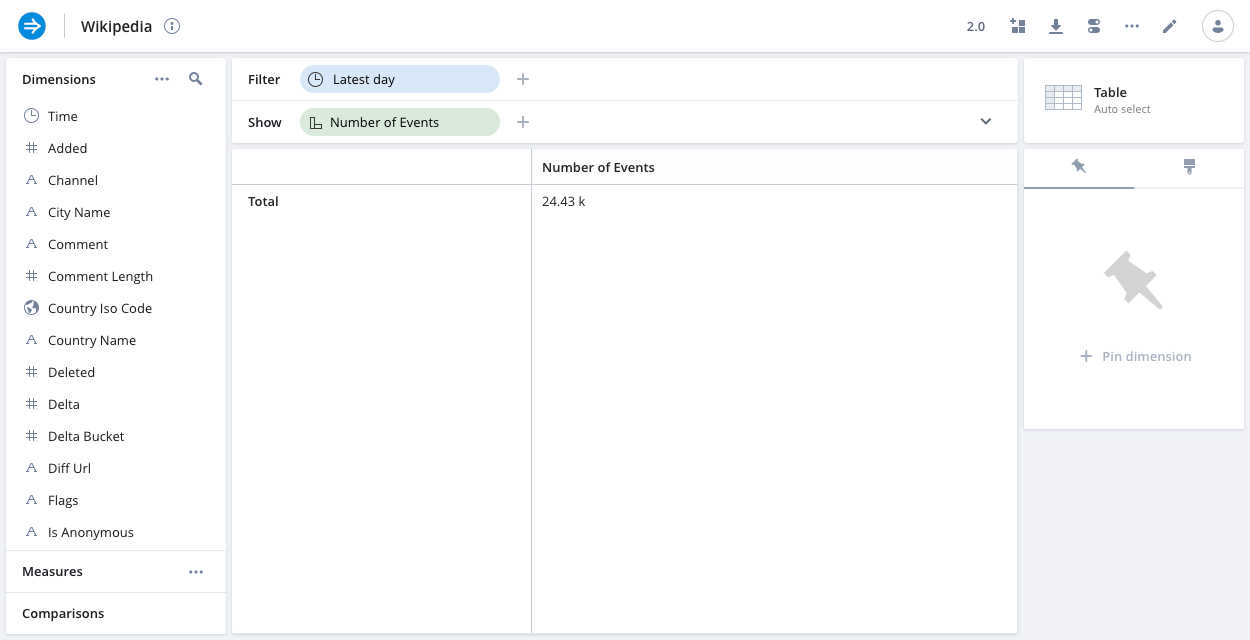You can edit them in the `Measures` tab of the data cube edit view: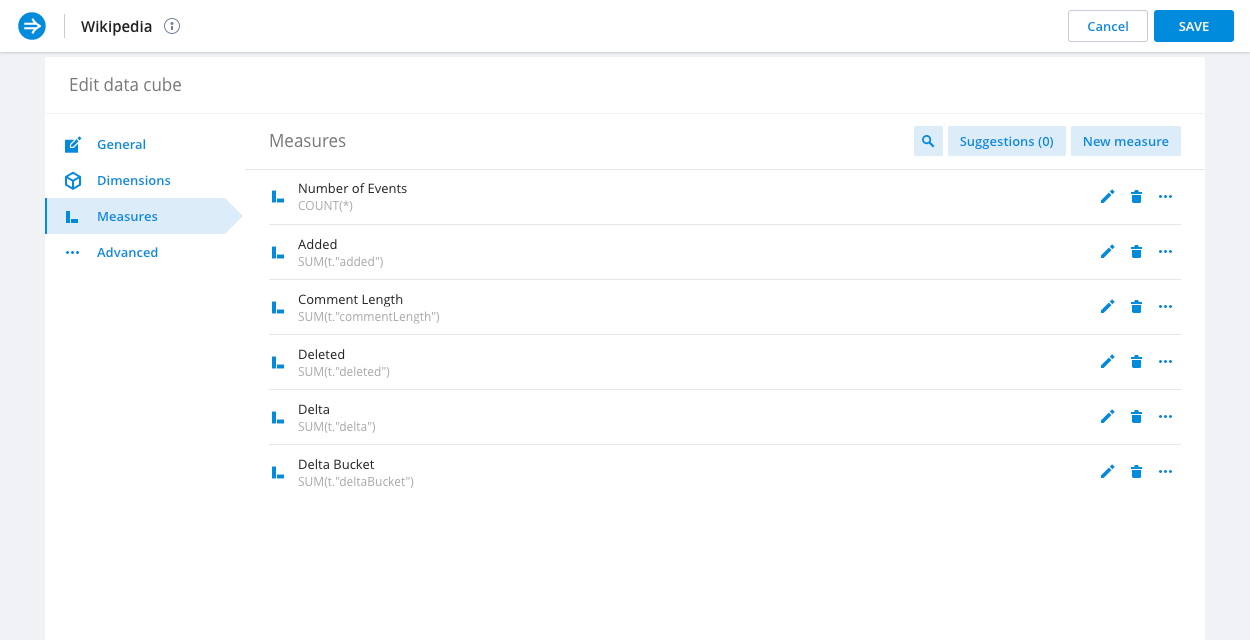## Measure groups​

You can group related measures into measure groups. This can be particularly useful for measures which come from the same basic attribute or are otherwise similar.

For example in a sales dataset scenario you might be interested in `Total revenue`, `Min revenue`, `Max revenue`, `Avg revenue`, `Median revenue per user`, and more. It might therefore make sense to group those measures.

To create a group simply click the `...` icon in one of the measures and select `Add to a new group`. Then drag the relevant measures into the newly created group.

## Measure formatting​

You can configure how a measure is formatted in the Format tab.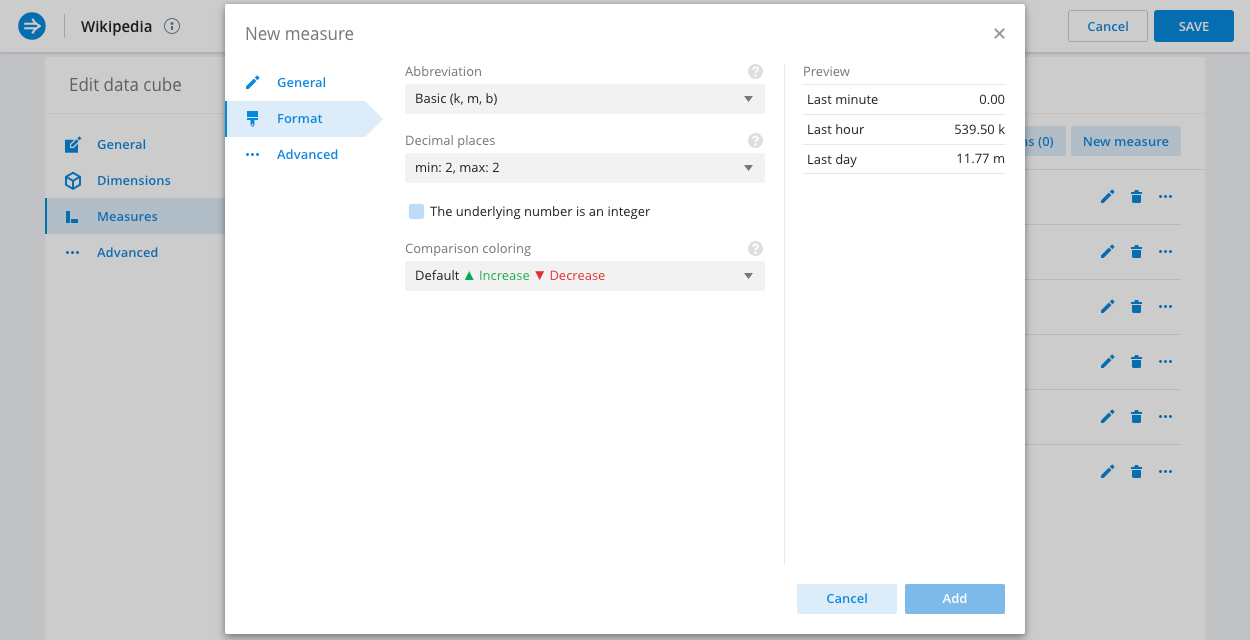Here you can select from a list of abbreviation sets that can be applied to format the measure, and adjust the decimal precision that will be displayed.

Additionally, when applying time comparisons to measure values, you can configure the display coloring for increased and decreased values.

## Measure fill options​

You can specify how to fill a measure on continuous visualizations (such as the line chart).

Data sets can have gaps in data, for example, due to down time for the systems that generate the data. There are a few ways to handle the missing data in charts, as determined by fill options. The following image shows four measures that are differentiated only by differing fill option.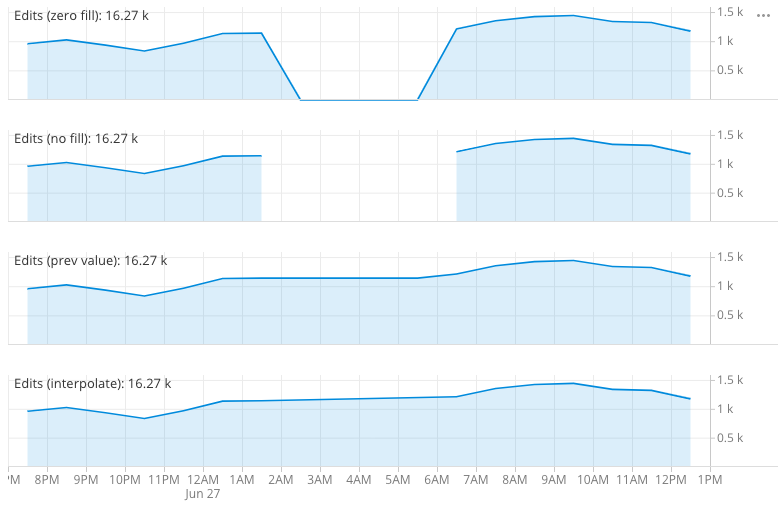The possible options are:

• Zero filled (default)fill missing data with zeros. This is most suitable if the measure represents an additive quantity.
• No fillleave missing data empty. This is suitable when missing values indicate that the data is not collected.
• Previous valuefill missing data with last value seen. This is suitable for sensor type data.
• Interpolate valuesinterpolate the missing data between the seen value. This is suitable for sensor type data.

## Measure transformations​

In the Advanced tab, you can transform a measure to be displayed as `Percent of parent segment` or as `Percent of total` instead of the default measure display.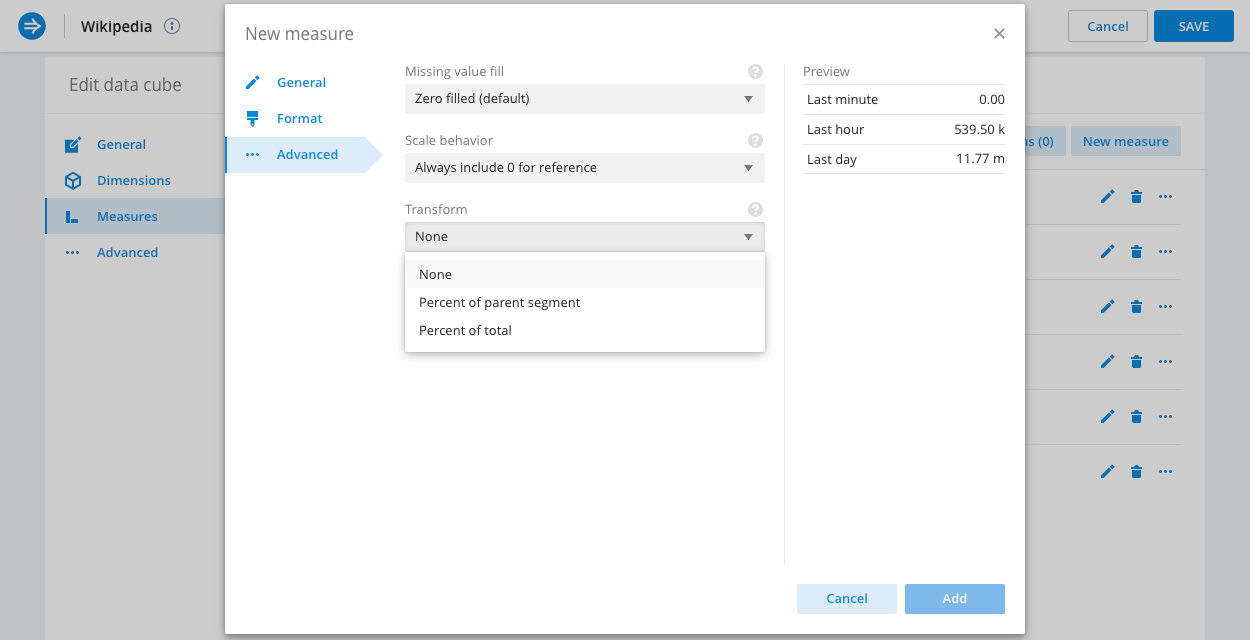## Create a measure​

You can create measures by clicking the New measure button in the Measures tab.

Use the Basic tab to configure simple measures consisting of a single aggregate function over a single column (with an optional filter).

A measure can also represent some post aggregation or computation (see the specific measure types section below). In that case you would use the Custom measure tab, where you can enter any supported Plywood or Pivot SQL expression as the measure's formula. See Custom dimensions and measures for more information.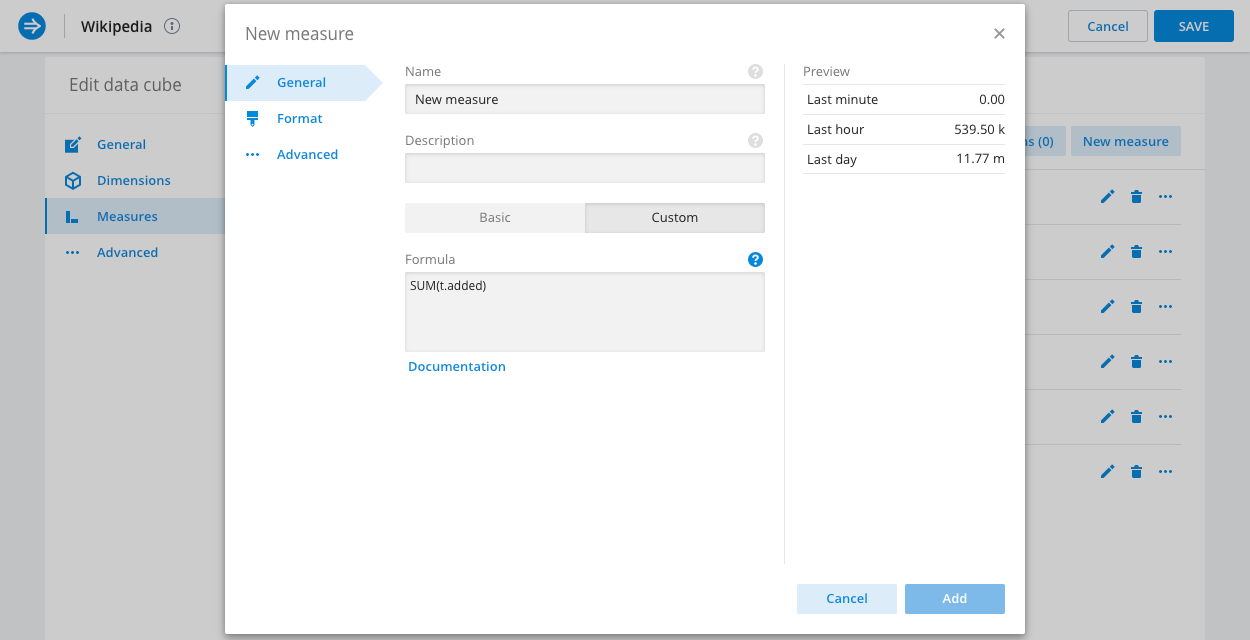## Measure suggestions​

Imply can provide suggestions about simple measures. This is done by scanning the schema of the underlying data source and automatically suggesting a measure, with the appropriate aggregate, for any column that is not already represented by one of the existing measures.

Measure suggestions are particularly useful if you have added a new column to your data source after creating the data cube and now want to represent it in your views.

## Custom measure examples​

In this section we will look at some of the many specific measure types supported.

The following examples show expressions as Pivot SQL and Plywood expressions.

### Filtered aggregations​

Filtered aggregations are very powerful. If, for example, your revenue in the US is a very important measure, you could express it as:

``SUM(t.revenue) FILTER (WHERE t.country = 'United States')``

It is also common to express a ratio of something filtered vs unfiltered.

``SUM(t.requests) FILTER (WHERE t.statusCode = 500) * 1.0 / SUM(t.requests)``

### Ratios​

Ratios are often useful to see the relationships in your data.

Here's one that expresses the computation of CPM:

``SUM(t.revenue) * 1.0 / SUM(t.impressions)``

### Quantiles​

A quantile can be a very useful measure of your data. For large datasets it is often more informative to look at the 98th or 99th quantile of the data rather than the max, as it filters out freak values. Similarly a median (or 50% quantile) can present different information than an average measure.

To add a quantile measure to your data simply define a formula like so:

``APPROX_QUANTILE_DS(t.revenue, 0.98)``

It is possible to fine-tune the accuracy of the approximate quantiles as well as pick different algorithm to use.

### Rates​

Sometimes the rate of change (over time) of a measure is very important.

There is a special function provided by Pivot `PIVOT_TIME_IN_INTERVAL('SECOND')` that gives the corresponding number of time units (first parameter) in the time interval over which the aggregation (whatever it is) is running.

Therefore it is possible to define a measure like

``SUM(t.bytes) / PIVOT_TIME_IN_INTERVAL('SECOND')``

to give you the accurate rate of bytes per second regardless of your filter window or selected split (hour, minute, or whatever).

### Nested aggregation measures​

It is possible to define measures that perform a sub-split and a sub aggregation as part of their overall aggregation. This is needed to express certain calculations which otherwise would not be possible.

The general form of a double aggregated measure's formula is:

``PIVOT_NESTED_AGG(SUB_SPLIT_EXPRESSION, INNER_AGGREGATE(...) AS "V", OUTER_AGGREGATE(t."V"))``

Where:

• `SUB_SPLIT_EXPRESSION` is the expression on which the data for this measure would be first split
• `INNER_AGGREGATE` is the aggregate that would be calculated for each bucket from the sub-split and will be assigned the name `V` (which is arbitrary)
• `OUTER_AGGREGATE` is the aggregate that will aggregate over the results of the inner aggregate, it should use the variable name declared above

Two examples of double aggregated measures are provided:

#### Netflow 95th percentile billing​

When dealing with netflow data at an ISP level one metric of interest is 5 minutely 95th percentile, which is used for burstable billing.

To add that as a measure (assuming there is a column called `bytes` which represents the bytes transferred during the interval), set the formula as follows:

``PIVOT_NESTED_AGG(TIME_FLOOR(t.__time, 'PT5M'), SUM(t.bytes) * 8.0 / 300 AS "B", APPROX_QUANTILE_DS(t."B", 0.95))``

Here the data is sub-split on 5 minute buckets (PT5M), then aggregated to calculate the bitrate (8 is the number of bits in a byte and 300 is the number of seconds in 5 minutes). Those inner 5 minute bitrates are then aggregated with the 95th percentile.

#### Average daily active users​

When looking at users engaging with a website, service, or app, we often need to know the number of daily active users.

This can be calculated as:

``PIVOT_NESTED_AGG(TIME_FLOOR(t.__time, 'P1D'), COUNT(DISTINCT t."user") AS "U", AVG(t."U"))``

Here the data is sub-split by day (P1D), and then an average is computed on top of that.

### Switching metric columns​

If you switch how you ingest your underlying metric and can't (or don't want to) recalculate all of the previous data, you could use a derived measure to seamlessly merge these two metrics in the UI.

Let's say you had a column called `revenue_in_dollars`, and for some reason you will now be ingesting it as `revenue_in_cents`.

Furthermore, right now your users are using the following measure:

``SUM(t.revenue_in_dollars)``

If your data had a 'clean break' where all events have either `revenue_in_dollars` or `revenue_in_cents` with no overlap, you could use:

``SUM(t.revenue_in_dollars) + SUM(t.revenue_in_cents) / 100``

If instead there was a period where you were ingesting both metrics, then the above solution would double count that interval. You can 'splice' these two metrics together at a specific time point.

Logically, you should be able to leverage Filtered aggregations to do:

``SUM(t.revenue_in_dollars) FILTER (WHERE t.__time < TIMESTAMP '2016-04-04 00:00:00')+ SUM(t.revenue_in_cents) FILTER (WHERE TIMESTAMP '2016-04-04 00:00:00' <= t.__time) / 100``

### Other custom measure examples​

Here are more example expressions that you can use to build custom measures:

Counts all the rows in the data cube.

``COUNT(*)``

Counts the distinct values of the dimension.

``COUNT(DISTINCT t."comment")``

Counts how many rows match a condition. This example counts how many rows in the dimension are true.

``SUM(CASE WHEN (t."dimension"='true') THEN 1 ELSE 0 END)``

Returns the sum of all values for the dimension.

``SUM(t."number_column")``

Returns the smallest value of the dimension.

``MIN(t."number_column")``

Returns the largest value of the dimension.

``MAX(t."number_column")``

Returns the average value of the dimension.

``AVG(t."number_column")``

Returns the x quantile of the dimension. You cannot use a compound expression in this function.

``APPROX_QUANTILE(t."dimension", 0.x)``

Limits the number of records to apply a given aggregate. This example returns x or fewer results.

``LIMIT (x)``

### Custom aggregations​

DEPRECATED: Custom aggregations are only possible in Plywood. You can no longer create new custom aggregations.